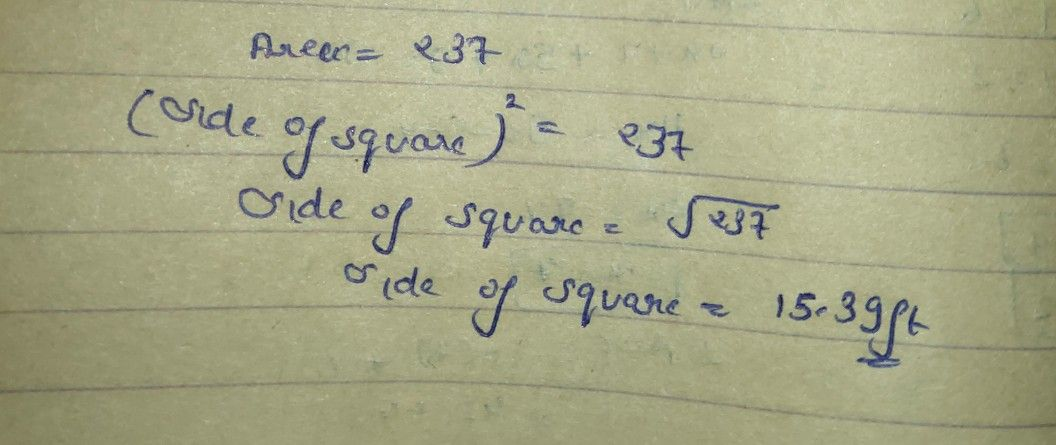Symbol
ProblemWhat $Iccx11$ Do Here is another activity hat will let you apply what you learned about eatitaating the quare root of a whole number in the nearest hundredths. Read and solve the following probl $111$ $1$ Ericka owns e square lot with a $411$ area 237 square $1x1$ To the nearest hundredths ot a foot, what is the side lengtth of the kitchen floor? 2. $Ac$ $7+1$ wants to use this tile to finish the floor in her room. The room $1$ square room and the area of the floor is $111$ square feet. She wants to put $8$ tiles along the edge of the floor. $1$ What is the length of one side of the room? b. How many tiles can she fit along one side? $3$ $1o11$ use one gallon of white paint to apply $11$ base coat $1$ paint on a square wall mural. The paint covers $380$ square feet per gallon. Estimate the $111x$ length $01$ the raural to the nearest hundredths of a foot.
7th-9th grade
Other
Search count: 107
SolutionQanda teacher - AskhinaStudent
thanks you